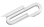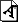Spring 2020

# Tue April 21

## 2D

First : let's go over any of the homework that you would like. We covered quite a lot in this 2D rotation stuff:

• center of mass
• rotational kinetic energy
• torque
• angular momentum

Discuss, and practice as needed.

Depending on how this feels, this week I'd like you to look into some of how the 3D stuff works.

## 3D

Second : what happens in the full 3D case?

The full answer is beyond the scope of this class. I am not sure yet how many problems we want to look at for this stuff. At least the basic precession equation, which is in the textbook.

The key point is that the torque, angular momentum, and spin vector all have both direction and length. And they can all point in different directions.

The definitions depend on what you decide is the coordinate system origin, similar to how the notion of potential energy depends on where you put zero.

For this class, the goal is to at least be exposed to a few specific iconic situations.

I am used to the explanations in chapter 7 of Kleppner - Kolenkow, particularly the off-axis baton, and examining the motion of two opposite points on a spinning wheel, and how their motion is changed by an applied force that tries to twist the wheel.

Discussion :

• Does a spinning marble falling towards the earth precess? Why or why not?
• The earth's axis precesses. What is causing the torque? (This one is not entirely obvious.)
• How can the spin axis and angular momentum axis be different?
• When a book is tossed into the air, it can "tumble" - the spin axis changes while in midair. Doesn't this violate some conservation law?
https://cs.marlboro.college /cours /spring2020 /mechanics /notes /apr21
## attachmentslast modified sizerotations.pdf Fri Dec 01 2023 07:16 pm 1.3M